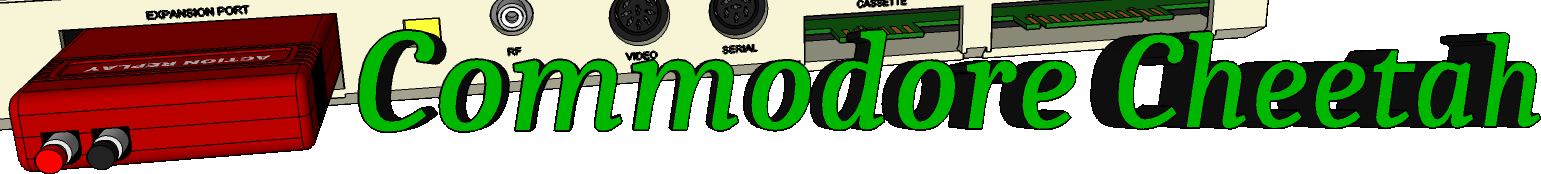Home PageeMail
email5-commodorecheetah.co.uk

Note: You will need to replace the '-' with the @ symbol.
This is to minimize spam.

 My BASIC Quick Reference Guide LET Abbreviation: L TYPE: Statement FORMAT: [LET] = Action: The LET statement can be used to assign a value to a variable. But the word LET is optional and therefore most advanced programmers leave LET out because it's always understood and wastes valuable memory. The equal sign (=) alone is sufficient when assigning the value of an expression to a variable name. EXAMPLES of LET Statement: 10 LET D= 12 (This is the same as D = 12) 20 LET E\$ = "ABC" 30 F\$ = "WORDS" 40 SUM\$= E\$ + F\$ (SUM\$ would equal ABCWORDS)

Commodore Cheetah made by Allen Monks, started in the year 2000.